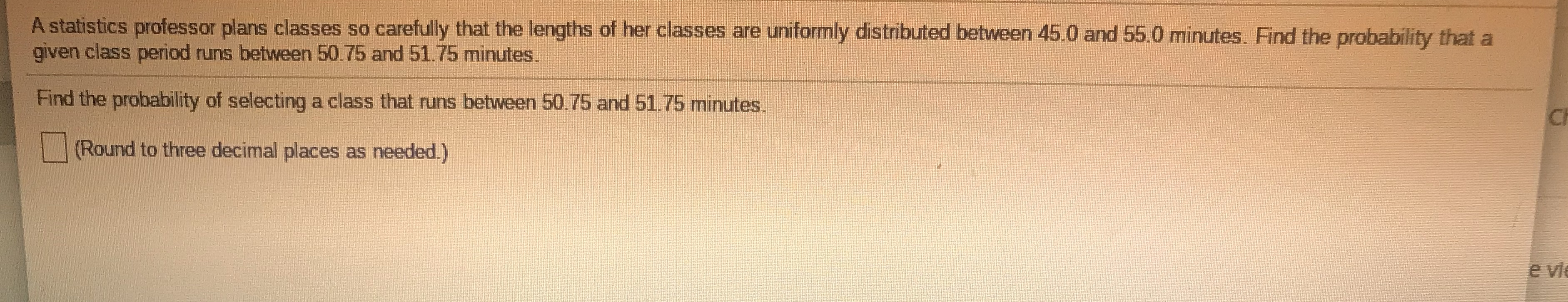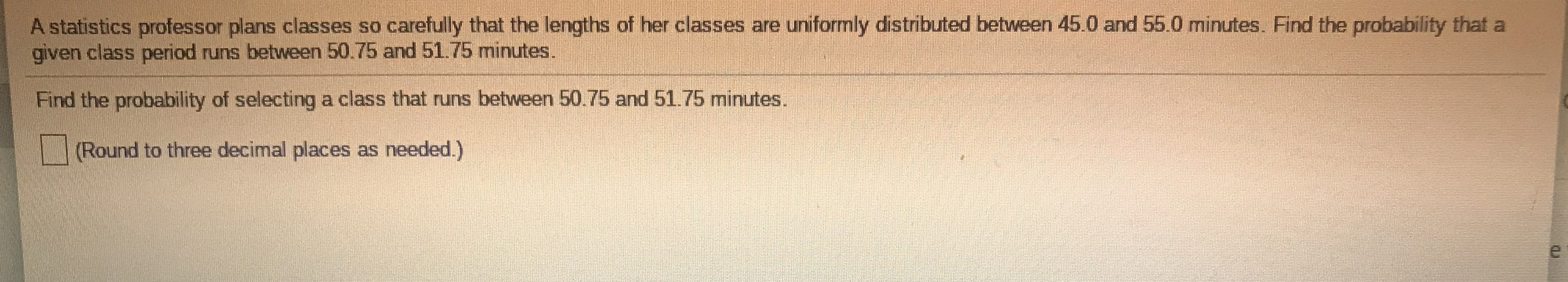# A statistics professor plans classes so carefully that the lengths of her classes are uniformly distributed between 45.0 and 55.0 minutes. Find the probability that a given class period runs between 50.75 and 51.75 minutes. Find the probability of selecting a class that runs between 50.75 and 51.75 minutes. (Round to three decimal places as needed.)

Questionfullscreenhelp_outlineImage TranscriptioncloseA statistics professor plans classes so carefully that the lengths of her classes are uniformly distributed between 45.0 and 55.0 minutes. Find the probability that a given class period runs between 50.75 and 51.75 minutes. Find the probability of selecting a class that runs between 50.75 and 51.75 minutes. (Round to three decimal places as needed.) fullscreen

### Want to see this answer and more?

Experts are waiting 24/7 to provide step-by-step solutions in as fast as 30 minutes!*

*Response times vary by subject and question complexity. Median response time is 34 minutes and may be longer for new subjects.
Tagged in
MathStatistics